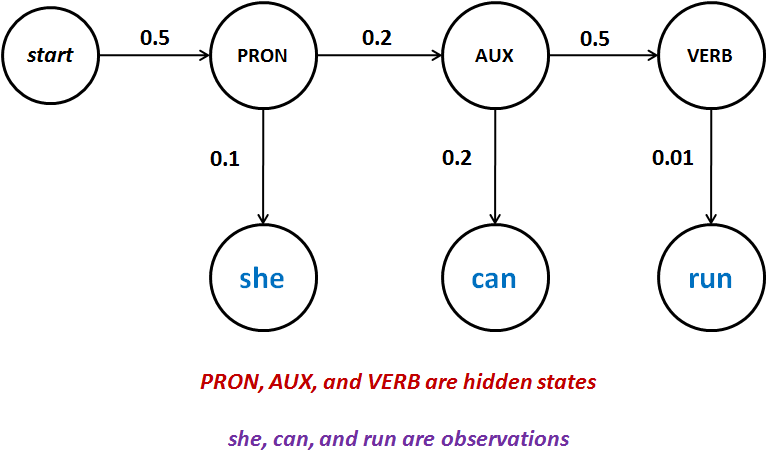Advanced Database Management System - Tutorials and Notes: POS tagging using HMM solved exercise

## POS Tagging using Hidden Markov Model - Solved Exercise

Question:
A Hidden Markov Model (HMM) is given in the table below;
 Transition probabilities Emission probabilities P(NOUN|PRON)=0.001 P(PRON|START)=0.5 P(VERB|AUX)=0.5 P(AUX|PRON)=0.2 P(NOUN|AUX)=0.001 P(VERB|NOUN)=0.2 P(NOUN|NOUN)=0.1 P(she|PRON)=0.1 P(run|VERB)=0.01 P(can|AUX)=0.2 P(can|NOUN)=0.001 P(run|NOUN)=0.001
Calculate the probability P(she|PRON can|AUX run|VERB). [ Or, calculate the probability P(she can run, PRON AUX VERB)]

Solution:
The following graph is extracted from the given HMM, to calculate the required probability;The probability of the given sentence can be calculated using the given bi-gram probabilities as follow;
P(she|PRON can|AUX run|VERB)
= P(PRON|START) * P(she|PRON) * P(AUX|PRON) * P(can|AUX) * P(VERB|AUX) * P(run|VERB)
= 0.5 * 0.1 * 0.2 * 0.2 * 0.5 * 0.01
= 0.00001
= 10-5
We arrived at this value by multiplying the transition and emission probabilities.

**********